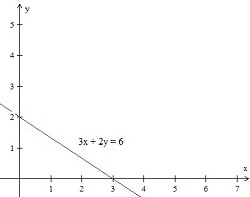# Graphing a line in quadrant 1 Online Quiz

Following quiz provides Multiple Choice Questions (MCQs) related to Graphing a line in quadrant 1. You will have to read all the given answers and click over the correct answer. If you are not sure about the answer then you can check the answer using Show Answer button. You can use Next Quiz button to check new set of questions in the quiz.### Explanation

Step 1:

Given equation 2x + y = 1; for y = 0, x = $\frac{1}{2}$; for x = 0, y = 1

Step 2:

Plotting the points and joining with a line we get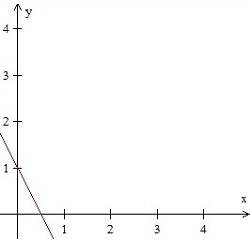### Explanation

Step 1:

Given equation 4x + y = 5; for y = 0, x = $\frac{5}{4}$; for x = 0, y = 5

Step 2:

Plotting the points and joining with a line we get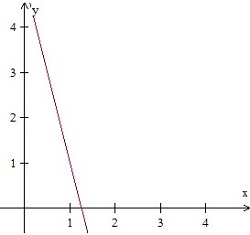### Explanation

Step 1:

Given equation 2x – y = 3; for y = 0, x = $\frac{3}{2}$; for x = 0, y = –3

Step 2:

Plotting the points and joining with a line we get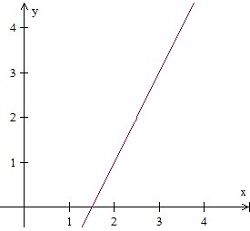### Explanation

Step 1:

Given equation x + 2y = 5; for y = 0, x = 5; for x = 0, y = $\frac{5}{2}$

Step 2:

Plotting the points and joining with a line we get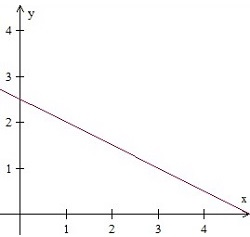### Explanation

Step 1:

Given equation 3x + y = 2; for y = 0, x = $\frac{2}{3}$; for x = 0, y = 2

Step 2:

Plotting the points and joining with a line we get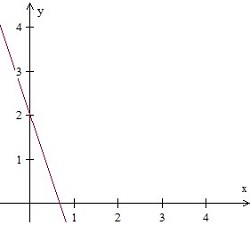### Explanation

Step 1:

Given equation 2x – y = 0; for y = 2, x = 1; for x = 0, y = 0

Step 2:

Plotting the points and joining with a line we get### Explanation

Step 1:

Given equation 3x + y = 7; for y = 0, x = $\frac{7}{3}$; for x = 0, y = 7

Step 2:

Plotting the points and joining with a line we get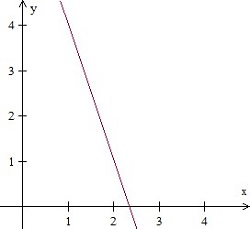### Explanation

Step 1:

Given equation 4x + y = 7; for y = 0, x = $\frac{7}{4}$; for x = 0, y = 7

Step 2:

Plotting the points and joining with a line we get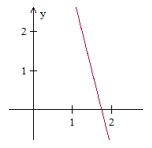### Explanation

Step 1:

Given equation x + 3y = 1; for y = 0, x = 1; for x = 0, y = $\frac{1}{3}$

Step 2:

Plotting the points and joining with a line we get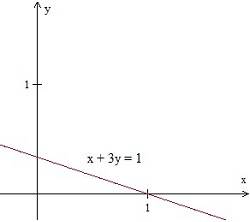### Explanation

Step 1:

Given equation 2x + 3y = 6; for y = 0, x = 3; for x = 0, y = 2

Step 2:

Plotting the points and joining with a line we get